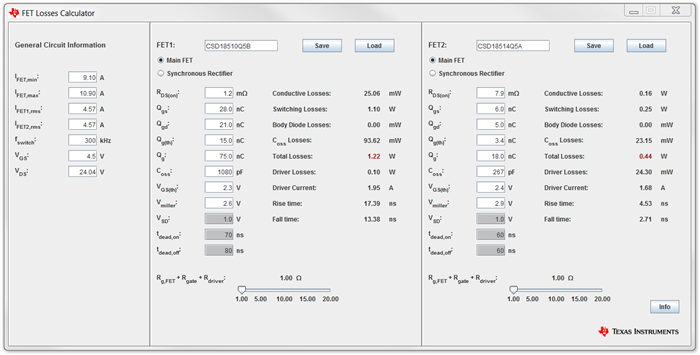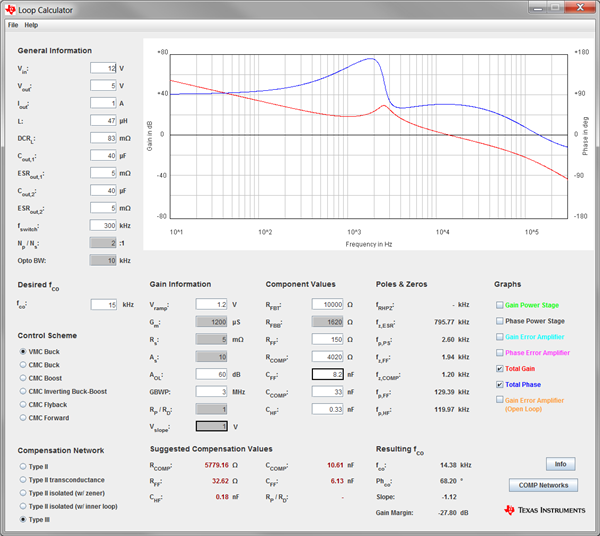# 10 more reasons to start using Power Stage Designer

Since 2011, TI’s Power Stage DesignerTM has been a great design aid for many electrical engineers when calculating the currents and voltages of different power-supply topologies. It is the easiest tool to start a new power-supply design, because all calculations are executed in real time and you get a direct feedback. With the evolution from version 3.0 to 4.0, Power Stage Designer offers three more topologies and a whole new set of features in the form of a toolbox that will help you speed up your power-supply designs even more.

The new toolbox contains a field-effect transistor (FET) losses calculator, a current-sharing calculator for parallel capacitors, an AC/DC bulk capacitor calculator, a resistor-capacitor (RC) snubber calculator for damping ringing across rectifiers, a resistor-capacitor-diode (RCD) snubber calculator for flyback converters, an output-voltage resistor divider calculator, dynamic analog and digital output voltage scaling calculators, a unit converter, and a Bode plotting tool for loop compensation. Let’s look at each of these 10 new features in detail.

No. 1: FET losses calculator

With this tool, you can easily compare different FETs that are either operating as a main switch or as a synchronous rectifier. The minimum, maximum and root mean square (RMS) current values, FET drain-source voltage and switching frequency will transfer from the chosen topology window. The tool can also help you assess the total metal-oxide semiconductor field-effect transistor (MOSFET) losses of synchronous converters after you’ve chosen applicable MOSFETs for the main switch and synchronous rectifier. Figure 1 shows the FET losses calculator window.Figure 1: FET losses calculator window

No. 2: Current-sharing calculator

When paralleling different kinds of capacitors at the input or output of a power converter, the capacitors experience different amounts of RMS current depending on their impedance. With Power Stage Designer, you can estimate the current stress for up to three parallel capacitors based on a first harmonic impedance model.

No. 3: AC/DC bulk capacitor calculator

AC-to-DC converters typically have a bulk capacitor behind the input rectifier to provide a quasi-constant input voltage to the power stage and the power-management controller. Power Stage Designer gives you a suggestion for the bulk capacitance based on different input parameters.

No. 4: RC snubber calculator for rectifiers

In power supplies, ringing across rectifiers can be a major issue if you need to pass electromagnetic interference (EMI) testing. There are different methods to deal with this problem and implementing an RC snubber network is an easy solution that might not require you to redesign your printed circuit board (PCB) layout. Power Stage Designer gives you an easy way to determine the starting values for your RC snubber network.

No. 5: RCD snubber calculator for flyback converters

Due to parasitics like transformer leakage inductance, flyback converters can experience voltage overshoot and ringing at the switching node. The easiest way to reduce the ringing and damping of the overshoot is to implement an RCD snubber circuit in parallel with the primary inductance of your flyback converter. Power Stage Designer can help you choose starting values for the snubber resistor and capacitor.

No. 6: Output-voltage resistor divider calculator

It is now possible to easily calculate the output-voltage feedback divider for your power supply based on output voltage, reference voltage and high-/low-side resistance, including tolerances, with Power Stage Designer.

No. 7: Dynamic analog output voltage scaling calculator

For some applications, the output voltage of a power converter needs to be adjustable in a certain output-voltage range. You can accomplish this by feeding a variable analog output voltage with a third resistor to the output voltage resistor divider. Power Stage Designer helps you find the values for the resistances needed based on the chosen output-voltage range, maximum adjusting voltage, reference voltage and the top feedback resistance.

No. 8: Dynamic digital output voltage scaling calculator

It’s also possible to adjust the output voltage of your power supply by paralleling multiple resistor/signal MOSFET combinations with the low-side feedback resistor. By enabling and disabling the MOSFETs with a microcontroller, it’s as if you’ve “programmed” different output voltages to the power supply. Power Stage Designer will assist you with choosing the resistance values for the feedback circuit.

No. 9: Unit converter

Power Stage Designer provides you with a little helper that converts different power-supply parameters, such as gain to factor or imperial to International System of Units (SI), and vice versa.

No. 10: Loop calculator

The loop calculator supports you by displaying Bode plots of the open- and closed-loop transfer functions for the voltage-mode control (VMC) buck and five different current-mode control (CMC) topologies – which are buck, boost, inverting buck-boost, forward and flyback. You can use five different compensation networks for closing the loop. Figure 2 shows the Power Stage Designer loop calculator window.Figure 2: Power Stage Designer loop calculator window

For the equations and assumptions behind the new toolbox, see the “Power Stage Designer User’s Guide.”

Additionally, Power Stage Designer 4.0 now supports a total of 20 topologies. The three new topologies in version 4.0 are:

• Series capacitor buck converter.
• Quasi-resonant/frequency-modulated flyback converter.
• Inductor-inductor-capacitor (LLC) half-bridge converter.

Figure 3 shows the topology window of the LLC half-bridge converter.Figure 3: Topology window of the LLC half-bridge converter

Get Power Stage Designer 4.0 and make your life as a power-supply designer a little bit easier.

Additional resources